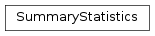# mvpa2.clfs.transerror.SummaryStatistics¶class `mvpa2.clfs.transerror.``SummaryStatistics`(targets=None, predictions=None, estimates=None, sets=None)

Basic class to collect targets/predictions and report summary statistics

It takes care about collecting the sets, which are just tuples (targets, predictions, estimates). While ‘computing’ the matrix, all sets are considered together. Children of the class are responsible for computation and display.

Attributes

 `error` `sets` `stats` `summaries` Return a list of separate summaries per each stored set

Methods

 `add`(targets, predictions[, estimates]) Add new results to the set of known results `as_string`([short, header, summary, description]) ‘Pretty print’ the matrix `compute`() Actually compute the confusion matrix based on all the sets `reset`() Cleans summary – all data/sets are wiped out

Initialize SummaryStatistics

targets or predictions cannot be provided alone (ie targets without predictions)

Parameters: targets Optional set of targets predictions Optional set of predictions estimates Optional set of estimates (which served for prediction) sets Optional list of sets

Attributes

 `error` `sets` `stats` `summaries` Return a list of separate summaries per each stored set

Methods

 `add`(targets, predictions[, estimates]) Add new results to the set of known results `as_string`([short, header, summary, description]) ‘Pretty print’ the matrix `compute`() Actually compute the confusion matrix based on all the sets `reset`() Cleans summary – all data/sets are wiped out
`add`(targets, predictions, estimates=None)

Add new results to the set of known results

`as_string`(short=False, header=True, summary=True, description=False)

‘Pretty print’ the matrix

Parameters: short : bool if True, ignores the rest of the parameters and provides consise 1 line summary header : bool print header of the table summary : bool print summary (accuracy) description : bool print verbose description of presented statistics
`compute`()

Actually compute the confusion matrix based on all the sets

`error`
`reset`()

Cleans summary – all data/sets are wiped out

`sets`
`stats`
`summaries`

Return a list of separate summaries per each stored set